Updating search results...

# 29 Results

View
Selected filters:
• MCCRS.Math.Practice.MP.7Only Sharing Permitted
CC BY-NC-ND
Rating
4,5 stars

يهدف هذا الدرس إلى مساعدة المعلمين على تقييم مدى نجاح الطلاب في تصوِّر المقاطع العرضية ثنائية الأبعاد الممثلة في الكائنات ثلاثية الأبعاد. يساعدك الدرس خصوصًا على تحديد الطلاب الذين يعانون من صعوبات في ادراك المقاطع العرضية ثنائية الأبعاد على طول النقاط المختلفة على مسطح ممثل في جسم ثلاثي الأبعاد ورسمها ومساعدة أولئك الطلاب.

نوع المادة:
التقييم
Lesson Plan
Provider:
Shell Center for Mathematical Education
Provider Set:
Mathematics Assessment Project (MAP)
04/26/2013Only Sharing Permitted
CC BY-NC-ND
Rating
0,0 stars

يهدف هذا الدرس لمساعدة المعلمين على تقييم مدى نجاح الطلاب في: العمل مع مفاهيم التطابق والتشابه، بما في ذلك تحديد الأضلاع المتناظرة والزوايا المتطابقة في المثلثات وبينها؛ وتحديد أهمية وجود المثال المضاد وفهمها؛ اثبات البراهين في سياق هندسي و تقييمها.

نوع المادة:
التقييم
Lesson Plan
Provider:
Shell Center for Mathematical Education
Provider Set:
Mathematics Assessment Project (MAP)
04/26/2013Only Sharing Permitted
CC BY-NC-ND
Rating
4,0 stars

يهدف هذا الدرس لمساعدتك في تقييم مدى نجاح الطلاب باستخدام الخصائص الهندسية من أجل حل المشاكل. على وجه الخصوص، سوف تدعمكم في تحديد ومساعدة الطلاب الذين لديهم الصعوبات التالية: حل المشاكل المتعلقة باستخدام قياسات الزوايا الداخلية للمضلعات؛ وحل المشاكل المتعلقة باستخدام قياسات الزوايا الخارجية للمضلعات.

نوع المادة:
التقييم
Lesson Plan
Provider:
Shell Center for Mathematical Education
Provider Set:
Mathematics Assessment Project (MAP)
04/26/2013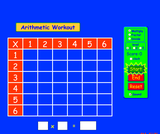Unrestricted Use
CC BY
Rating
5,0 stars

Brush up on your multiplication, division, and factoring skills with this interactive multiplication chart. Three levels and timed or untimed options are available.

نوع المادة:
محاكاة
Provider:
Provider Set:
المحاكاة التفاعلية لموقع PhET
المؤلف:
Michael Dubson
11/05/2011Only Sharing Permitted
CC BY-NC-ND
Rating
3,0 stars

يهدف هذا الدرس لمساعدة المعلمين على تقييم مدى نجاح الطلاب في إنشاء المعادلات الخطية وحلها. على وجه الخصوص، يساعدك الدرس على تحديد الطلاب الذين لديهم الصعوبات الآتية ومساعدتهم: حل عادلات بمتغير واحد وحل المعادلات الخطية بأكثر من طريقة واحدة.

نوع المادة:
التقييم
Lesson Plan
Provider:
Shell Center for Mathematical Education
Provider Set:
Mathematics Assessment Project (MAP)
04/26/2013Only Sharing Permitted
CC BY-NC-ND
Rating
0,0 stars

This lesson unit is intended to help teachers assess how well students are able to interpret exponential and linear functions and in particular to identify and help students who have the following difficulties: translating between descriptive, algebraic and tabular data, and graphical representation of the functions; recognizing how, and why, a quantity changes per unit intervale; and to achieve these goals students work on simple and compound interest problems.

نوع المادة:
التقييم
Lesson Plan
Provider:
Shell Center for Mathematical Education
Provider Set:
Mathematics Assessment Project (MAP)
04/26/2013Only Sharing Permitted
CC BY-NC-ND
Rating
3,0 stars

This lesson unit is intended to help teachers assess how well students are able to: use the Pythagorean theorem to derive the equation of a circle; and translate between the geometric features of circles and their equations.

نوع المادة:
التقييم
Lesson Plan
Provider:
Shell Center for Mathematical Education
Provider Set:
Mathematics Assessment Project (MAP)
04/26/2013Only Sharing Permitted
CC BY-NC-ND
Rating
0,0 stars

This lesson unit is intended to help teachers assess how well students are able to: translate between the equations of circles and their geometric features; and sketch a circle from its equation.

نوع المادة:
التقييم
Lesson Plan
Provider:
Shell Center for Mathematical Education
Provider Set:
Mathematics Assessment Project (MAP)
04/26/2013Only Sharing Permitted
CC BY-NC-ND
Rating
4,0 stars

This lesson unit is intended to help teachers assess how well students are able to: estimate lengths of everyday objects; convert between decimal and scientific notation; and make comparisons of the size of numbers expressed in both decimal and scientific notation.

نوع المادة:
التقييم
Lesson Plan
Provider:
Shell Center for Mathematical Education
Provider Set:
Mathematics Assessment Project (MAP)
04/26/2013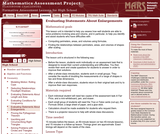Only Sharing Permitted
CC BY-NC-ND
Rating
0,0 stars

This lesson unit is intended to help teachers assess how well students are able to solve problems involving area and volume, and in particular, to help you identify and assist students who have difficulties with the following: computing perimeters, areas and volumes using formulas; and finding the relationships between perimeters, areas, and volumes of shapes after scaling.

نوع المادة:
التقييم
Lesson Plan
Provider:
Shell Center for Mathematical Education
Provider Set:
Mathematics Assessment Project (MAP)
04/26/2013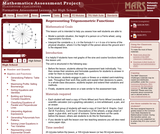Only Sharing Permitted
CC BY-NC-ND
Rating
0,0 stars

This lesson unit is intended to help teachers assess how well students are able to: model a periodic situation, the height of a person on a Ferris wheel, using trigonometric functions; and interpret the constants a, b, c in the formula h = a + b cos ct in terms of the physical situation, where h is the height of the person above the ground and t is the elapsed time.

نوع المادة:
التقييم
Lesson Plan
Provider:
Shell Center for Mathematical Education
Provider Set:
Mathematics Assessment Project (MAP)
04/26/2013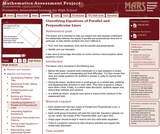Only Sharing Permitted
CC BY-NC-ND
Rating
0,0 stars

This lesson unit is intended to help teachers assess how well students are able to understand the relationship between the slopes of parallel and perpendicular lines and, in particular, to help identify students who find it difficult to: find, from their equations, lines that are parallel and perpendicular; and identify and use intercepts. It also aims to encourage discussion on some common misconceptions about equations of lines.

نوع المادة:
التقييم
Lesson Plan
Provider:
Shell Center for Mathematical Education
Provider Set:
Mathematics Assessment Project (MAP)
04/26/2013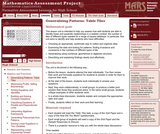Only Sharing Permitted
CC BY-NC-ND
Rating
0,0 stars

This lesson unit is intended to help teachers assess how well students are able to identify linear and quadratic relationships in a realistic context: the number of tiles of different types that are needed for a range of square tabletops. In particular, this unit aims to identify and help students who have difficulties with: choosing an appropriate, systematic way to collect and organize data; examining the data and looking for patterns; finding invariance and covariance in the numbers of different types of tile; generalizing using numerical, geometrical or algebraic structure; and describing and explaining findings clearly and effectively.

نوع المادة:
التقييم
Lesson Plan
Provider:
Shell Center for Mathematical Education
Provider Set:
Mathematics Assessment Project (MAP)
04/26/2013Only Sharing Permitted
CC BY-NC-ND
Rating
3,0 stars

This lesson unit is intended to help you assess how well students working with square numbers are able to: choose an appropriate, systematic way to collect and organize data, examining the data for patterns; describe and explain findings clearly and effectively; generalize using numerical, geometrical, graphical and/or algebraic structure; and explain why certain results are possible/impossible, moving towards a proof.

نوع المادة:
التقييم
Lesson Plan
Provider:
Shell Center for Mathematical Education
Provider Set:
Mathematics Assessment Project (MAP)
04/26/2013Only Sharing Permitted
CC BY-NC-ND
Rating
0,0 stars

This lesson unit is intended to help teachers assess how well students are able to use geometric properties to solve problems. In particular, the lesson will help you identify and help students who have the following difficulties: solving problems by determining the lengths of the sides in right triangles; and finding the measurements of shapes by decomposing complex shapes into simpler ones. The lesson unit will also help students to recognize that there may be different approaches to geometrical problems, and to understand the relative strengths and weaknesses of those approaches.

نوع المادة:
التقييم
Lesson Plan
Provider:
Shell Center for Mathematical Education
Provider Set:
Mathematics Assessment Project (MAP)
04/26/2013Only Sharing Permitted
CC BY-NC-ND
Rating
4,0 stars

This lesson unit is intended to help teachers assess how well students are able to interpret percent increase and decrease, and in particular, to identify and help students who have the following difficulties: translating between percents, decimals, and fractions; representing percent increase and decrease as multiplication; and recognizing the relationship between increases and decreases.

نوع المادة:
التقييم
Lesson Plan
Provider:
Shell Center for Mathematical Education
Provider Set:
Mathematics Assessment Project (MAP)
04/26/2013Only Sharing Permitted
CC BY-NC-ND
Rating
0,0 stars

This lesson unit is intended to help teachers assess how well students are able to use geometric properties to solve problems. In particular, it will help you identify and help students who have difficulty: decomposing complex shapes into simpler ones in order to solve a problem; bringing together several geometric concepts to solve a problem; and finding the relationship between radii of inscribed and circumscribed circles of right triangles.

نوع المادة:
التقييم
Lesson Plan
Provider:
Shell Center for Mathematical Education
Provider Set:
Mathematics Assessment Project (MAP)
04/26/2013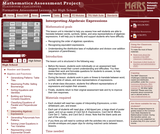Only Sharing Permitted
CC BY-NC-ND
Rating
0,0 stars

This lesson unit is intended to help teachers assess how well students are able to translate between words, symbols, tables, and area representations of algebraic expressions. It will help teachers to identify and support students who have difficulty in: recognizing the order of algebraic operations; recognizing equivalent expressions; and understanding the distributive laws of multiplication and division over addition (expansion of parentheses).

نوع المادة:
التقييم
Lesson Plan
Provider:
Shell Center for Mathematical Education
Provider Set:
Mathematics Assessment Project (MAP)
04/26/2013Only Sharing Permitted
CC BY-NC-ND
Rating
4,0 stars

This lesson unit is intended to help you assess how well students are able to: Perform arithmetic operations, including those involving whole-number exponents, recognizing and applying the conventional order of operations; Write and evaluate numerical expressions from diagrammatic representations and be able to identify equivalent expressions; apply the distributive and commutative properties appropriately; and use the method for finding areas of compound rectangles.

نوع المادة:
التقييم
Lesson Plan
Provider:
Shell Center for Mathematical Education
Provider Set:
Mathematics Assessment Project (MAP)
04/26/2013Only Sharing Permitted
CC BY-NC-ND
Rating
5,0 stars

This lesson unit is intended to help teahcers assess how well students are able to interpret speed as the slope of a linear graph and translate between the equation of a line and its graphical representation.

نوع المادة:
التقييم
Lesson Plan
Provider:
Shell Center for Mathematical Education
Provider Set:
Mathematics Assessment Project (MAP)